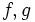# Translation surface

This article defines a property that makes sense for a surface embedded in$\R^3$, viz three-dimensional Euclidean space. The property is invariant under orthogonal transformations and scaling, i.e., under all similarity transformations.
A surface in$\R^3$ is termed a translation surface if it has the following Cartesian equation:$z = f(x) + g(y)$
where$f,g$ are smooth functions from open sets in$\R$, to$\R$.